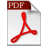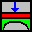Axisymmetric Structures and Tanks
ELPLA (ELastic PLAte) is a software which is used for analyzing geotechnical and structure problems. One of them is analyzing axisymmetric structure or tank with the real subsoil model.

• Three known subsoil models for the analysis of axisymmetric structures and tanks (standard models) are considered. The subsoil models are the Simple Assumption Model, the Winkler Model and the Continuum Model
• The mathematical solution of the structures is based on the FE-Method. The program can analyze different types of subsoil models, especially the Continuum model that considers any number of soil layers under the structure base
• A good advantage of this program is the capability to handle the three analyses of flexible, elastic and rigid bases. In addition, the mesh of the rigid and flexible bases can be constructed to be analogous to the finite elements mesh of the elastic foundation. Therefore, the three analyses can be compared easily and correctly
DocumentsAnalysis of Axisymmetric Structures and Tanks CatalogueAnalysis of Axisymmetric Structures and Tanks by ELPLA Part I (Numerical models)Analysis of Axisymmetric Structures and Tanks by ELPLA Part II (Verification Examples)Analysis of Axisymmetric Structures and Tanks by ELPLA Part III (Tutorial Examples)
DataELPLA-Data Files of Verification ExamplesELPLA-Data Files of Tutorial Examples
Features
• User interface and help system are available in 3 languages: English, German and Arabic
• Analyzing a cylindrical tank considering the wall and the base as one unit on layered soil
• Analyzing axisymmetric structures with or without opening base using annular finite elements
• Analysis of an elastic, a rigid or a flexible base on layered soil
• Numerical model of soil-structure interaction is under 9 calculation methods
• Automatic generation for the FE mesh of the wall and the base
• Powerful mesh generator using templates for different axisymmetric structure shapes
• Translational and rotational springs can be added at nodes
• Elastic or fixed rotations and deflections can be taken into account.
• Determining contact pressures, settlements, internal forces, subgrade reactions
• Node coordinates and boundary nodes of the FE mesh can be imported from a table via MS Excel
• Spherical or flat roof of the axisymmetric structures and tanks are also possible
• Variable wall and base thickness
• Consideration of reduction coefficients α according to DIN4019 Part 1
• The soil is defined by a boring log has multi-layers with different soil material
• Drawing soil layers by different symbols and colors according to DIN 4023 for easy identification
• Consideration of groundwater and overburden pressure effects
• Color representation of the dimensions and results on the screen or printer
• Presentation of the results in the plan or diagrams
• Presentation of the data, element groups slab thickness, boundary conditions, loads, … in the plan
• Distribution of results on the wall and the base
• Tabulation of data and final results on the screen or printer
• Results can be saved in an ASCII file
• The drawings can optionally be saved as a WMF file
• There are detailed explanations with numerical examples in three books are available
• Short help information can be requested at any interface location
• Import or export the data to MS Excel
• Export the results and diagrams to MS Excel
• Export the data and results to MS Word
• Copying drawings to the clipboard for use in word processors
• Import or export the FE-Net as DXF format
• This textbook describes procedures and methods available in ELPLA to analyze circular cylindrical shells structures
• Circular cylindrical tank resting on any layered compressible soil is considered as one unit taking into account the soil-structure interaction effect
• The purpose of this text is to present the methods, equations, procedures, and techniques used in the formulation and development of the ELPLA function for analyzing tanks on different subsoil models
• An understanding of these concepts will be of great benefit in applying the software, resolving difficulties and judging the acceptability of the results
• Two familiar types of subsoil models are considered, Winkler’s model and Continuum model. In addition, the simple assumption model is also considered. This model assumes linear contact pressure on the base of the tank
• The mathematical solution of the circular cylindrical tanks is based on the Finite Element Method using axi-symmetric circular cylindrical shell elements In which, axi-symmetric shell finite elements represent the tank wall and tank base according to the nature geometry of the structureAnalysis of Axisymmetric Structures and Tanks by ELPLA Part I (Numerical models)
• ELPLA can analyze different structures with different types of subsoil models
• In this textbook some problems published previously by researchers using different methods of analyses and models are compared with the results obtained by the analysis used in this textbook using ELPLA
• A verification study is carried out using ELPLA to analyze circular cylindrical tanks with different subsoil models
• The mathematical solution of the circular cylindrical tanks is based on the Finite Element Method using circular cylindrical shell elements
• Items to be checked under different conditions are internal forces, deformations and rotations in the tank wall and baseAnalysis of Axisymmetric Structures and Tanks by ELPLA Part II (Verification Examples)ELPLA-Data Files of Verification Examples